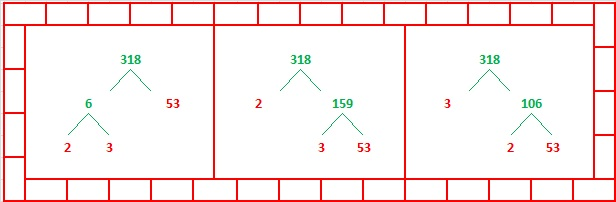# 318 Christmas Factor Tree Gif

• 318 is a composite number.
• Prime factorization: 318 = 2 x 3 x 53
• The exponents in the prime factorization are 1, 1, and 1. Adding one to each and multiplying we get (1 + 1)(1 + 1)(1 + 1) = 2 x 2 x 2 = 8. Therefore 318 has exactly 8 factors.
• Factors of 318: 1, 2, 3, 6, 53, 106, 159, 318
• Factor pairs: 318 = 1 x 318, 2 x 159, 3 x 106, or 6 x 53
• 318 has no square factors that allow its square root to be simplified. √318 ≈ 17.833This factoring tree gif solves and completes this Level ONE puzzle right before your eyes. Scroll down to see three possible factor trees for the number 318.make animated gifs like this at MakeAGifPrint the puzzles or type the factors on this excel file: 10 Factors 2014-12-08

1.Steve Morris